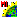# NEARNEGH.SML

###Download

#### Syntax Highlighing:

```comments, key words, predefined symbols, class members & methods, functions & classes
```
```#************************************************************************
#The following code updates the two (previously defined)
#nearest neighbor databases:
# NEARNEGH.SML
# AUTHOR:  Paul Pope, Environmental Monitoring Program, UW-Madison
#
# This SML script updates the nearest
# neighbor databases for the polygons of a vector object.
# The following database tables must be defined before
# running the script:
#
# Table Name:  Num_NN
# Description:  The number of nearest neighbors for each polygon
# Number of Fields:  1
# Field: Number_Neighbors
# Field Type:  Integer (Width: 10, SLong)
# Attachment Type:  One Record Per Element
#
# Table Name:  NN
# Description:  The polygon number of the nearest neighbors for each polygon
# Number of Fields:  1
# Field Type:  Integer (Width: 10, SLong)
# Attachment Type:  No Restrictions
#
clear();
# Prompt the user to enter a vector object
vector V;
GetInputVector(V);
numeric number_of_polygons = NumVectorPolys(V);
# Loop over all the polygons in the vector object
numeric polygon_number;
for polygon_number = 1 to number_of_polygons step 1
{
#   print(" ")
#   print("Working on polgon number = ", polygon_number)
array numeric line_list;
array numeric nearest_neighbor_list;
numeric number_of_lines = GetVectorPolyLineList(V, line_list, polygon_number);
#  print("Number of Lines = ", number_of_lines)
# Loop over all the lines which make up the current polygon
numeric k;
numeric number_of_neighbors = 0;
numeric leftpoly, rightpoly, outside_polygon;
numeric line_number, flag;
for line_number = 1 to number_of_lines step 1
{
#     print("Line Number = ", line_list[line_number])
leftpoly = V.line[line_list[line_number]].Internal.LeftPoly;
rightpoly = V.line[line_list[line_number]].Internal.RightPoly;
#     print("Left Poly = ", leftpoly, "Right Poly = ", rightpoly)
# The polygon which is not the current polygon
# is a neighbor polygon
if (leftpoly != polygon_number) then
{
outside_polygon = leftpoly;
}
if (rightpoly != polygon_number) then
{
outside_polygon = rightpoly;
}
#     print("Outside polygon = ", outside_polygon)
# Ensure that the neighbor polygon is not already
# in the nearest neighbor list
flag = 0;
for k = 1 to number_of_neighbors step 1
{
if (nearest_neighbor_list[k] == outside_polygon) then
{
flag = 1;
}
}
# If the neighbor polygon is not already in
# the nearest neighbor list, add it to the list
if (flag != 1) then
{
# Make a copy of the nearest neighbor list
if (number_of_neighbors > 0) then
{
array numeric temp_list[number_of_neighbors];
for k = 1 to number_of_neighbors step 1
{
temp_list[k] = nearest_neighbor_list[k];
}
}
# Enlarge the nearest neighbor list to include
# another entry and add the nearest neighbor to
# the end of the list
number_of_neighbors = number_of_neighbors + 1;
array numeric nearest_neighbor_list[number_of_neighbors];
nearest_neighbor_list[number_of_neighbors] = outside_polygon;
# Copy the previous nearest neighbors to the
# newly expanded list
if (number_of_neighbors > 1) then
{
for k = 1 to (number_of_neighbors - 1) step 1
{
nearest_neighbor_list[k] = temp_list[k];
}
}
#        print("Nearest Neighbor = ", outside_polygon)
}  # End of flag if statement
}  # End of looping over the number of lines
# Update the nearest neighbor polygon database tables
#   print("For polygon number = ", polygon_number,", ")
#   print("the number of nearest neighbors = ", number_of_neighbors)
V.poly[polygon_number].Num_NN.Number_NN = number_of_neighbors;
for k = 1 to number_of_neighbors step 1
{
#     print("Nearest Neighbor[",k,"] = ", nearest_neighbor_list[k])
V.poly[polygon_number].NN[k].NearestNeighbor = nearest_neighbor_list[k];
}
}  # End of looping over the number of polygons
# End of SML script```